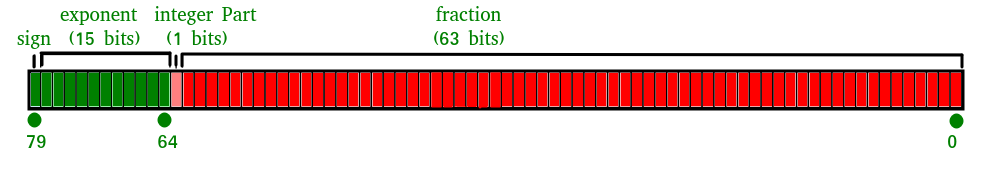# JavaScript Number isSafeInteger() Method

• Last Updated : 24 Dec, 2021

Below is the example of the Number isSafeInteger() Method.

• Example:

## Javascript

 ` `

• Output:
`Output : true`

What is a Safe Integer?
A safe integer is an integer which has the following properties

• A number which can be represented as an IEEE-754 double precision number i.e. all integers from (253 – 1) to -(253 – 1)).

What is an IEEE-754 double-precision number?
Double-precision floating-point format is a computer number format, which occupies 64 bits in computer memory.
It represents a wide range of numeric values by using a floating-point.
The IEEE 754 standard specifies a binary64 as having:
Sign bit: 1 bit
Exponent: 11 bits
Significand precision: 53 bits (52 explicitly stored)isSafeInteger() Method In JavaScript

The isSafeInteger() method in JavaScript is used to check whether a number is a safe integer or not.

Syntax:

`Number.isSafeInteger(Value)`

Parameters Used:
1. Value: It is the number to be check for safeinteger() method.

Return Value:
The toExponential() method in JavaScript returns true if the value is a safe integer Number, else it returns false.

Examples:

```Input : Number.isSafeInteger(23)
Output : true

Input : Number.isSafeInteger(-23)
Output : true

Input : Number.isSafeInteger(0.5)
Output : false

Input : Number.isSafeInteger(0/0)
Output : false```

1. Passing a positive number as an argument in the isSafeInteger() method.

## Javascript

 ` `

Output:

`Output : true`

2. Passing a negative number as an argument in the isSafeInteger() method.

## Javascript

 ` `

Output:

`Output : true`

3. Passing a number(with decimals) as an argument in the isSafeInteger() method.

## Javascript

 ` `

Output:

`Output : false`

4. Passing an equation( which equates to an infinite value) as an argument in the isSafeInteger() method.

## Javascript

 ` `

Output:

`Output : false`

Code Explanation: JavaScript uses double-precision floating-point format numbers as specified in IEEE 754 and can only safely represent numbers between -(253 – 1) and 253 – 1.If the parameter passed lies in this specified range then the number.isSafeInteger() method returns true else false.

Supported Browsers:

• Google Chrome 34 and above
• Firefox 32 and above
• Apple Safari 10 and above
• Opera 21 and above
• Edge 12 and above

My Personal Notes arrow_drop_up
Recommended Articles
Page :